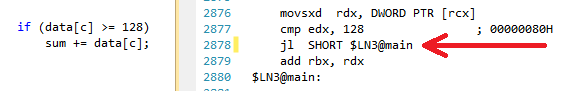+1
0

## 代码执行的效率

#### 第一个例子

PHP中Getter和Setter的效率来源reddit

```<?php
//dog_naive.php

class dog {
public \$name = "";
public function setName(\$name) {
\$this-&gt;name = \$name;
}
public function getName() {
return \$this-&gt;name;
}
}

\$rover = new dog();
//通过Getter/Setter方式
for (\$x=0; \$x<10; \$x++) {
\$t = microtime(true);
for (\$i=0; \$i<1000000; \$i++) {
\$rover->setName("rover");
\$n = \$rover->getName();
}
echo microtime(true) - \$t;
echo "\n";
}
//直接存取变量方式
for (\$x=0; \$x<10; \$x++) {
\$t = microtime(true);
for(\$i=0; \$i<1000000; \$i++) {
\$rover->name = "rover";
\$n = \$rover->name;
}
echo microtime(true) - \$t;
echo "\n";
}
?>```

```class dog {
private \$_name = "";
function __set(\$property,\$value) {
if(\$property == 'name') \$this->_name = \$value;
}
function __get(\$property) {
if(\$property == 'name') return \$this->_name;
}
}```

#### 第二个例子

```def main():
for i in xrange(10**8):
pass
main()```

```for i in xrange(10**8):
pass```

```13 FOR_ITER                 6 (to 22)
16 STORE_FAST               1 (i)
19 JUMP_ABSOLUTE           13```
```13 FOR_ITER                 6 (to 22)
16 STORE_NAME               1 (i)
19 JUMP_ABSOLUTE           13```

（注：在C/C++中，这个不是一个问题）

#### 第三个例子

``` for (unsigned i = 0; i < 100000; ++i) {
// primary loop
for (unsigned j = 0; j < arraySize; ++j) {
if (data[j] >= 128)
sum += data[j];
}
}```Image by Mecanismo, from Wikimedia Commons:http://commons.wikimedia.org/wiki/File:Entroncamento_do_Transpraia.JPG```T = 走分支（条件表达式为true）
N = 不走分支(条件表达式为false)

data[] = 0, 1, 2, 3, 4, ... 126, 127, 128, 129, 130, ... 250, 251, 252, ...
branch = N  N  N  N  N  ...   N    N    T    T    T  ...   T    T    T  ...

= NNNNNNNNNNNN ... NNNNNNNTTTTTTTTT ... TTTTTTTTTT  (easy to predict)```
```data[] = 226, 185, 125, 158, 198, 144, 217, 79, 202, 118,  14, 150, 177, 182, 133, ...
branch =   T,   T,   N,   T,   T,   T,   T,  N,   T,   N,   N,   T,   T,   T,   N  ...

= TTNTTTTNTNNTTTN ...   (completely random - hard to predict)```

```if (data[j] >= 128)
sum += data[j];
```

```int t = (data[j] - 128) >> 31;
sum += ~t & data[j];```

“没有分叉”的性能基本上和“排好序有分支”一个样，无论是C/C++，还是Java。

（全文完）

（转载本站文章请注明作者和出处酷壳 – CoolShell.cn，请勿用于任何商业用途）

————————============感谢42qu.com为本站提供VPS============————————
 您可能也喜欢：Why C++ ? 王者归来GCC 用 C++ 来编译PHP分页技术的代码和示例如何写出无法维护的代码22个开源的PHP框架 无觅

### 评价列表(1)

• +1 guest voted at 2012-07-24 15:29:05